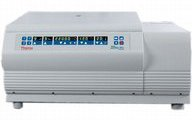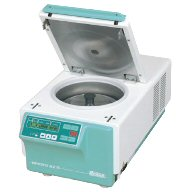# Centrifuge RPM and RCF Calculator

This calculator allows you to inter-convert between RPM and RCF for any centrifuge with a known rotor size. There is a limited list of rotors below for manual rotor selection.

Variable to solve for Value Units
Rotational speed RPM
G-force (RCF) xg

You are currently solving for rotational speed (RPM)

## Instructions

1) Choose what to solve for. Use the radio boxes in the table above to select what you wish to solve for. e.g. if you know the radius and the desired G-force, select RPM to solve for. That box will then be disabled and will be used to display the answer.

2) Enter the known values. Use the two active boxes in the table to enter your known values. Use the units listed in the right hand column.

3) The answer is displayed. The answer is calculated as you type and displayed in blue text in the relevant box. To begin another calculation, just type in the boxes or select a new variable to solve for.

## How the calculator works

The calculator solves unknown values using the formula:

g = (1.118 x 10-5) x R x S2

Where g is the relative centrifugal force, R is the radius of the rotor in centimetres and S is the speed of the rotor in revolutions per minute.

## Limitations of the calculator

Rounding: The calculator rounds all given answers to integers. Values ending in .5 or higher are rounded up to 1. Values that end lower than .5 are rounded down. This means that there is a small degree of imprecision in displayed results.

Note that the calculator only rounds the numbers at the end of the calculation, just before display. All previous steps use the full precision numbers. Also, it is possible to enter non-integers to the calculator and these will be treated correctly but any answers generated will be rounded.

Average rotor size: The calculator uses only one rotor size and does not attempt to calculate maximum G-force or average G-force for a given rotor. The exact G-force experienced by samples in a centrifuge depends on the shape of the rotor and the placement of the samples within it.

## Manual centrifuge rotor selection

Here is a list of centrifuges in common use where I work. It is here for ease of use for people who work with these centrifuges.

 Centrifuge Rotor Rotor ID Rotor radius (mm) Maximum speed (rpm) Select this rotor Sorvall legend RT24x microcentrifuge tubes 3332 87 170004x Microplate carriers (4x 500g) 7500 6449 159 4600Hettich Mikro 22R24x microcentrifuge tubes 1195-L 87 1600012x 35 gram tubes 1015 87 6000First published on 21st September 2008 and last modified on 25th June 2011.